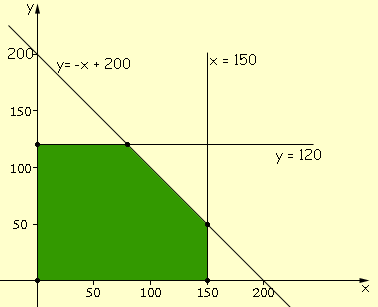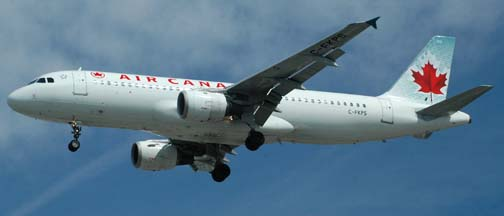Natasha Glydon

Consider this scenario: your school is planning to make toques and mitts to sell at the winter festival as a fundraiser.  The school’s sewing classes divide into two groups – one group can make toques, the other group knows how to make mitts.  The sewing teachers are also willing to help out.  Considering the number of people available and time constraints due to classes, only 150 toques and 120 pairs of mitts can be made each week.  Enough material is delivered to the school every Monday morning to make a total of 200 items per week.  Because the material is being donated by community members, each toque sold makes a profit of \$2 and each pair of mitts sold makes a profit of \$5.

In order to make the most money from the fundraiser, how many of each item should be made each week?  It is important to understand that profit (the amount of money made from the fundraiser) is equal to the revenue (the total amount of money made) minus the costs: Proft = Revenue - Cost. Because the students are donating their time and the community is donating the material, the cost of making the toques and mitts is zero.  So in this case, profitrevenue.

If the quantity you want to optimize (here, profit) and the constraint conditions (more on them later) are linear, then the problem can be solved using a special organization called linear programming.  Linear programming enables industries and companies to find optimal solutions to economic decisions.  Generally, this means maximizing profits and minimizing costs.  Linear programming is most commonly seen in operations research because it provides a “best” solution, while considering all the constraints of the situation.  Constraints are limitations, and may suggest, for example, how much of a certain item can be made or in how much time.

Creating equations, or inequalities, and graphing them can help solve simple linear programming problems, like the one above.  We can assign variables to represent the information in the above problem.

x = the number of toques made weekly
y = the number of pairs of mitts made weekly

Then, we can write linear inequalities based on the constraints from the problem.

 x ≤ 150 and y ≤ 120 The students can only make up to 150 toques and up to 120 pairs of mitts each week. This is one restriction. x + y ≤ 200 The total number of mitts and toques made each week cannot exceed 200.  This is the material restriction.

We may also want to consider that x ≥ 0 and y ≥ 0. This means that we cannot make -3 toques.

Our final equation comes from the goal of the problem.  We want to maximize the total profit from the toques and mitts.  This can be represented by \$2x + \$5y = P, where P is the total profit, since there are no costs in production.  If the school sells x toques, then they make \$2x from the sales of toques.  If the school sells y mitts, then they make \$5y from the sales of mitts.

In some applications, the linear equations are very complex with numerous constraints and there are too many variables to work out manually, so they have special computers and software to perform the calculations efficiently.  Sometimes, linear programming problems can be solved using matrices or by using an elimination or substitution method, which are common strategies for solving systems of linear equations.

Using the equations and inequations generated above, we can graph these, to find a feasible region.  Our feasible region is the convex polygon that satisfies all of the constraints.  In this situation, one of the vertices of this polygon will provide the optimal choice, so we must first consider all of the corner points of the polygon and find which pair of coordinates makes us the most money.  From our toque and mitt example, we can produce the following graph:We can see that our feasible region (the green area) has vertices of (0, 120), (150, 0),
(150, 50), and (80, 120).  By substituting these values for x and y in our revenue equation, we can find the optimal solution.

R = 2x + 5y
R = 2(80) + 5(120)
R = \$760

After considering all of the options, we can conclude that this is our maximum revenue.  Therefore, the sewing students (and teachers) must make 80 toques and 120 pairs of mitts each week in order to make the most money.  We can check that these solutions satisfy all of our restrictions:
80 + 120 ≤ 200. This is true.  We know that we will have enough material to make 80 toques and 120 pairs of mitts each week.  We can also see that our values for x and y are less than 150 and 120, respectively.  So, not only is our solution possible, but it is the best combination to optimize profits for the school.  This is a fairly simple problem, but it is easy to see how this type of organization can be useful and very practical in the industrial world.

Airlines

The airline industry uses linear programming to optimize profits and minimize expenses in their business.  Initially, airlines charged the same price for any seat on the aircraft.  In order to make money, they decided to charge different fares for different seats and promoted different prices depending on how early you bought your ticket.  This required some linear programming.  Airlines needed to consider how many people would be willing to pay a higher price for a ticket if they were able to book their flight at the last minute and have substantial flexibility in their schedule and flight times.  The airline also needed to know how many people would only purchase a low price ticket, without an in-flight meal.  Through linear programming, airlines were able to find the optimal breakdown of how many tickets to sell at which price, including various prices in between.Image reproduced with permission of Goleta Air & Space Museum

Airlines also need to consider plane routes, pilot schedules, direct and in-direct flights, and layovers.  There are certain standards that require pilots to sleep for so many hours and to have so many days rest before flying.  Airlines want to maximize the amount of time that their pilots are in the air, as well.  Pilots have certain specializations, as not all pilots are able to fly the same planes, so this also becomes a factor.  The most controllable factor an airline has is its pilot’s salary, so it is important that airlines use their optimization teams to keep this expense as low as possible.  Because all of these constraints must be considered when making economic decisions about the airline, linear programming becomes a crucial job.

The Manufacturing Industry
Many other industries rely on linear programming to enhance the economy of their business.  These include:
• The military
• Capital budgeting
• Designing diets
• Conservation of resources
• Economic growth prediction
• Transportation systems (busses, trains, etc.)
• Strategic games (e.g. chess)
• Factory manufacturing

All of these industries rely on the intricate mathematics of linear programming.  Even farmers use linear programming to increase the revenue of their operations, like what to grow, how much of it, and what to use it for.  Amusement parks use linear programming to make decisions about queue lines.  Linear programming is an important part of operations research and continues to make the world more economically efficient.